# 目录：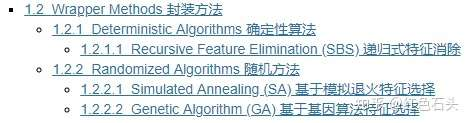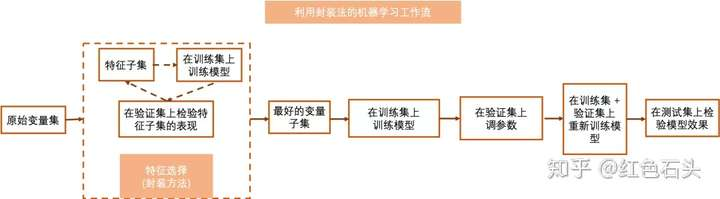### 1.2.1 Deterministic Algorithms 确定性算法

#### 1.2.1.1 Recursive Feature Elimination (SBS) 递归式特征消除

# RFE函数 演示
import numpy as np
from sklearn.feature_selection import RFE

# 直接载入数据集
from sklearn.datasets import fetch_california_housing
dataset = fetch_california_housing()
X, y = dataset.data, dataset.target # 利用 california_housing 数据集来演示

# 选择前15000个观测点作为训练集
# 剩下的作为测试集
train_set = X[0:15000,:]
test_set = X[15000:,]
train_y = y[0:15000]

# 选择用于衡量子集表现的有监督的机器学习模型
from sklearn.ensemble import ExtraTreesRegressor # 使用ExtraTrees 模型作为示范
clf = ExtraTreesRegressor(n_estimators=25)
selector = RFE(estimator = clf, n_features_to_select = 4, step = 1)
# 与RFECV不同，此处RFE函数需要用户定义选择的变量数量，此处设置为选择4个最好的变量，每一步我们仅删除一个变量

selector = selector.fit(train_set, train_y) # 在训练集上训练

transformed_train = train_set[:,selector.support_]  # 转换训练集
assert np.array_equal(transformed_train, train_set[:,[0,5,6,7]]) # 选择了第一个，第六个，第七个及第八个变量

transformed_test = test_set[:,selector.support_] # 转换训练集
assert np.array_equal(transformed_test, test_set[:,[0,5,6,7]]) # 选择了第一个，第六个，第七个及第八个变量

# RFECV 函数 演示
import numpy as np
from sklearn.feature_selection import RFECV

# 直接载入数据集
from sklearn.datasets import fetch_california_housing
dataset = fetch_california_housing()
X, y = dataset.data, dataset.target # 利用 california_housing 数据集来演示

# 选择前15000个观测点作为训练集
# 剩下的作为测试集
train_set = X[0:15000,:]
test_set = X[15000:,]
train_y = y[0:15000]

# 选择用于衡量子集表现的有监督的机器学习模型
from sklearn.ensemble import ExtraTreesRegressor # 使用ExtraTrees 模型作为示范
clf = ExtraTreesRegressor(n_estimators=25)
selector = RFECV(estimator = clf, step = 1, cv = 5) # 使用5折交叉验证
# 每一步我们仅删除一个变量
selector = selector.fit(train_set, train_y)

transformed_train = train_set[:,selector.support_]  # 转换训练集
assert np.array_equal(transformed_train, train_set) # 选择了所有的变量

transformed_test = test_set[:,selector.support_] # 转换训练集
assert np.array_equal(transformed_test, test_set) # 选择了所有的变量


### 1.2.2 Randomized Algorithms 随机方法

#### 1.2.2.1 Simulated Annealing (SA) 基于模拟退火特征选择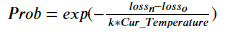Prob为接受表现不佳的特征子集的概率， ????? 为新特征子集的损失（loss）， ????? 为新特征子集创建前的最优（最低）损失（loss）， ???_??????????? 为当前的温度。模拟退火的伪代码为: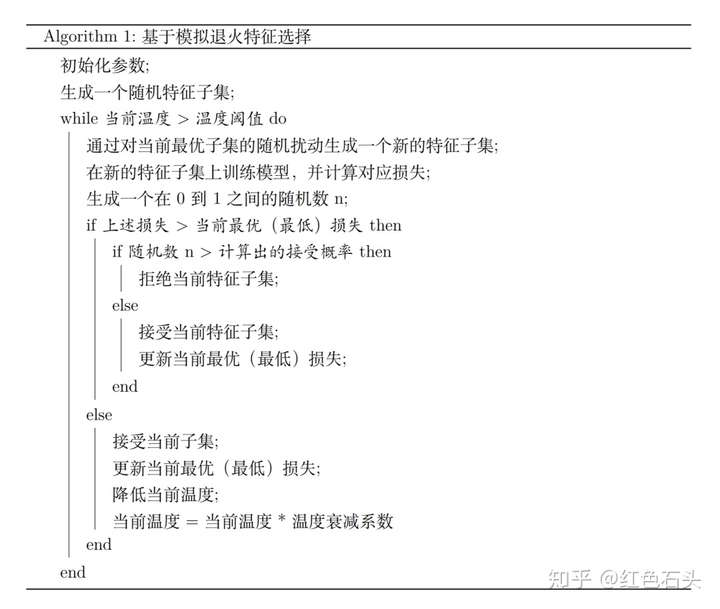import sys
sys.path.append("..")
from SA import Simulated_Annealing # 导入我们撰写的模块

# 直接载入数据集
from sklearn.datasets import fetch_california_housing
dataset = fetch_california_housing()
X, y = dataset.data, dataset.target # 利用 california_housing 数据集来演示

# 选择前15000个观测点作为训练集
# 剩下的作为测试集
train_set = X[0:15000,:]
test_set = X[15000:,]
train_y = y[0:15000]

# 选择用于衡量子集表现的有监督的机器学习模型
from sklearn.ensemble import ExtraTreesRegressor # 使用ExtraTrees 模型作为示范

# 选择模拟退火中评价特征子集的的损失函数
from sklearn.metrics import mean_squared_error # 回归问题我们使用MSE

clf = ExtraTreesRegressor(n_estimators=25)
selector = Simulated_Annealing(loss_func = mean_squared_error, estimator = clf,
init_temp = 0.2, min_temp = 0.005, iteration = 10, alpha = 0.9)
# 在训练集中训练
# SA.py中有具体每个参数的含义，此处不赘述

selector.fit(X_train = train_set, y_train = train_y, cv = 5) # 使用5折交叉验证

transformed_train = selector.transform(train_set) # 转换训练集
transformed_test = selector.transform(test_set)  # 转换测试集

selector.best_sol # 返回最优特征的索引
selector.best_loss; # 返回最优特征子集对应的损失


import sys
sys.path.append("..")
import numpy as np
import random
from SA import Simulated_Annealing # 导入我们撰写的模块

from sklearn.datasets import load_iris  # 利用iris数据作为演示数据集

# 载入数据集
X, y = iris.data, iris.target

# iris 数据集使用前需要被打乱顺序
np.random.seed(1234)
idx = np.random.permutation(len(X))
X = X[idx]
y = y[idx]

# 选择前100个观测点作为训练集
# 剩下的前20个观测点作为验证集，剩下的30个观测作为测试集
train_set = X[0:100,:]
val_set = X[100:120,:]
test_set = X[120:,:]

train_y = y[0:100]
val_y = y[100:120]
test_y = y[120:]

# 重制随机种子
# 随机方法需要随机性的存在
random.seed()
np.random.seed()

# 选择用于衡量子集表现的有监督的机器学习模型
from sklearn.ensemble import ExtraTreesClassifier # we use extratree as predictive model

# 选择模拟退火中评价特征子集的的损失函数
from sklearn.metrics import log_loss # 回归问题中，我们使用交叉熵损失函数

clf = ExtraTreesClassifier(n_estimators=25)
selector = Simulated_Annealing(loss_func = log_loss, estimator = clf,
init_temp = 0.2, min_temp = 0.005, iteration = 10,
alpha = 0.9, predict_type = 'predict_proba')
# 在训练集中训练
# SA.py中有具体每个参数的含义，此处不赘述

selector.fit(X_train = train_set, y_train = train_y, X_val = val_set,
y_val = val_y, stop_point = 15)
# 此函数允许用户导入自己定义的验证集，此处尝试一下

transformed_train = selector.transform(train_set)  # 转换训练集
transformed_test = selector.transform(test_set)  # 转换测试集

selector.best_sol # 返回最优特征的索引
selector.best_loss; # 返回最优特征子集对应的损失


#### 1.2.2.2 Genetic Algorithm (GA) 基于基因算法特征选择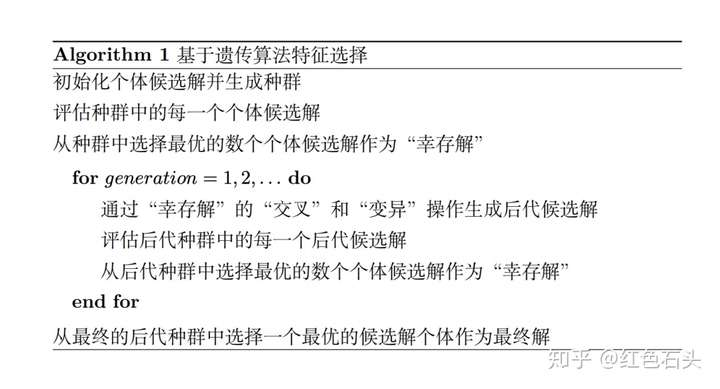import sys
sys.path.append("..")
from GA import Genetic_Algorithm # 导入我们撰写的模块

# 直接载入数据集
from sklearn.datasets import fetch_california_housing
dataset = fetch_california_housing()
X, y = dataset.data, dataset.target # 利用 california_housing 数据集来演示

# 选择前15000个观测点作为训练集
# 剩下的作为测试集
train_set = X[0:15000,:]
test_set = X[15000:,]
train_y = y[0:15000]

# 选择用于衡量子集表现的有监督的机器学习模型
from sklearn.ensemble import ExtraTreesRegressor # 使用ExtraTrees 模型作为示范

# 选择模拟退火中评价特征子集的的损失函数
from sklearn.metrics import mean_squared_error # 回归问题我们使用MSE

clf = ExtraTreesRegressor(n_estimators=25)
selector = Genetic_Algorithm(loss_func = mean_squared_error, estimator = clf,
n_gen = 10, n_pop = 20, algorithm = 'NSGA2')
# 在训练集中训练
# GA.py中有具体每个参数的含义，此处不赘述

selector.fit(X_train = train_set, y_train = train_y, cv = 5) # 使用5折交叉验证

transformed_train = selector.transform(train_set) # 转换训练集
transformed_test = selector.transform(test_set)  # 转换测试集

selector.best_sol # 返回最优特征的索引
selector.best_loss; # 返回最优特征子集对应的损失


import sys
sys.path.append("..")
import numpy as np
import random
from GA import Genetic_Algorithm # 导入我们撰写的模块

from sklearn.datasets import load_iris  # 利用iris数据作为演示数据集

# 载入数据集
X, y = iris.data, iris.target

# iris 数据集使用前需要被打乱顺序
np.random.seed(1234)
idx = np.random.permutation(len(X))
X = X[idx]
y = y[idx]

# 选择前100个观测点作为训练集
# 剩下的前20个观测点作为验证集，剩下的30个观测作为测试集
train_set = X[0:100,:]
val_set = X[100:120,:]
test_set = X[120:,:]

train_y = y[0:100]
val_y = y[100:120]
test_y = y[120:]

# 重制随机种子
# 随机方法需要随机性的存在
random.seed()
np.random.seed()

# 选择用于衡量子集表现的有监督的机器学习模型
from sklearn.ensemble import ExtraTreesClassifier # we use extratree as predictive model

# 选择模拟退火中评价特征子集的的损失函数
from sklearn.metrics import log_loss # 回归问题中，我们使用交叉熵损失函数

clf = ExtraTreesClassifier(n_estimators=25)
selector = Genetic_Algorithm(loss_func = log_loss, estimator = clf,
n_gen = 15, n_pop = 10, predict_type = 'predict_proba')
# 在训练集中训练
# GA.py中有具体每个参数的含义，此处不赘述

selector.fit(X_train = train_set, y_train = train_y, X_val = val_set,
y_val = val_y, stop_point = 15)
# 此函数允许用户导入自己定义的验证集，此处尝试一下

transformed_train = selector.transform(train_set)  # 转换训练集
transformed_test = selector.transform(test_set)  # 转换测试集

selector.best_sol # 返回最优特征的索引
selector.best_loss; # 返回最优特征子集对应的损失### 觉得文章有用就打赏一下文章作者

#### 支付宝扫一扫打赏#### 微信扫一扫打赏